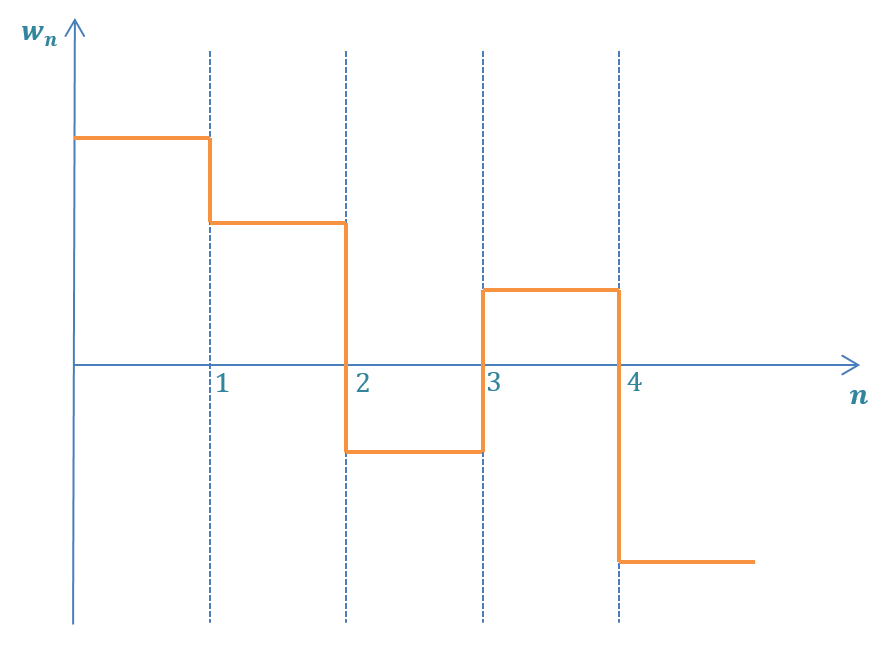﻿ Covariance Extrapolation Equation

# Covariance Extrapolation Equation

I assume the reader is already familiar with the concept of covariance extrapolation (prediction). We've already met the Covariance Extrapolation Equation (or Predictor Covariance Equation) in the "One-dimensional Kalman Filter" section. In this section, we derive the Kalman Filter Covariance Extrapolation Equation in matrix notation.

The general form of the Covariance Extrapolation Equation is given by:

$\boldsymbol{P}_{n+1,n} = \boldsymbol{FP}_{n,n}\boldsymbol{F}^{T} + \boldsymbol{Q}$
Where:
 $$\boldsymbol{P}_{n,n}$$ is the squared uncertainty of an estimate (covariance matrix) of the current state $$\boldsymbol{P}_{n+1,n}$$ is the squared uncertainty of a prediction (covariance matrix) for the next state $$\boldsymbol{F}$$ is the state transition matrix that we derived in the "Modeling linear dynamic systems" section $$\boldsymbol{Q}$$ is the process noise matrix

## The estimate covariance without process noise

Let's assume that the process noise is equal to zero $$(Q=0)$$, then:

$\boldsymbol{P}_{n+1,n} = \boldsymbol{FP}_{n,n}\boldsymbol{F}^{T}$

The derivation is relatively straightforward. I've shown in the "Essential background II" section, that:

$COV(\boldsymbol{x}) = E \left( \left( \boldsymbol{x - \mu_{x}} \right) \left( \boldsymbol{x - \mu_{x}} \right)^{T} \right)$

Where vector $$x$$ is a system state vector.

Therefore:

$\boldsymbol{P}_{n,n} = E \left( \left( \boldsymbol{\hat{x}_{n,n} - \mu_{x_{n,n}}} \right) \left( \boldsymbol{\hat{x}_{n,n} - \mu_{x_{n,n}}} \right)^{T} \right)$

According to the state extrapolation equation:

$\boldsymbol{\hat{x}}_{n+1,n} = \boldsymbol{F\hat{x}}_{n,n} + \boldsymbol{G\hat{u}}_{n,n}$

Therefore:

$\boldsymbol{P}_{n+1,n} = E \left( \left( \boldsymbol{\hat{x}}_{n+1,n} - \boldsymbol{\mu}_{x_{n+1,n}} \right) \left( \boldsymbol{\hat{x}}_{n+1,n} - \boldsymbol{\mu}_{x_{n+1,n}} \right)^{T} \right) =$

$= E \left( \left( \boldsymbol{F\hat{x}}_{n,n} + \boldsymbol{G\hat{u}}_{n,n} - \boldsymbol{F\mu_{x}}_{n,n} - \boldsymbol{G\hat{u}}_{n,n} \right) \left( \boldsymbol{F\hat{x}}_{n,n} + \boldsymbol{G\hat{u}}_{n,n} - \boldsymbol{F\mu_{x}}_{n,n} - \boldsymbol{G\hat{u}}_{n,n} \right)^{T} \right) =$

$= E \left( \boldsymbol{F} \left( \boldsymbol{\hat{x}}_{n,n} - \boldsymbol{\mu}_{x_{n,n}} \right) \left( \boldsymbol{F} \left( \boldsymbol{\hat{x}}_{n,n} - \boldsymbol{\mu}_{x_{n,n}} \right) \right)^{T} \right) =$

Apply the matrix transpose property: $$\boldsymbol{(AB)}^T = \boldsymbol{B}^T \boldsymbol{A}^T$$

$= E \left(\boldsymbol{F} \left( \boldsymbol{\hat{x}}_{n,n} - \boldsymbol{\mu}_{x_{n,n}} \right) \left( \boldsymbol{\hat{x}}_{n,n} - \boldsymbol{\mu}_{x_{n,n}} \right)^{T} \boldsymbol{F}^{T} \right) =$

$= \boldsymbol{F} E \left( \left( \boldsymbol{\hat{x}_{n,n} - \mu_{x_{n,n}}} \right) \left( \boldsymbol{\hat{x}_{n,n} - \mu_{x_{n,n}}} \right)^{T} \right) \boldsymbol{F}^{T} =$

$= \boldsymbol{F} \boldsymbol{P}_{n,n} \boldsymbol{F}^{T}$

## Constructing the process noise matrix $$Q$$

As you already know, the system dynamics is described by:

$\boldsymbol{\hat{x}}_{n+1,n} = \boldsymbol{F\hat{x}}_{n,n} + \boldsymbol{G\hat{u}}_{n,n} + \boldsymbol{w}_{n}$

Where $$\boldsymbol{w}_{n}$$ is the process noise at the time step $$n$$.

We've discussed the process noise and its influence on the Kalman Filter performance in the "One-dimensional Kalman Filter" section. In the one-dimensional Kalman Filter, the process noise variance is denoted by $$q$$.

In the multidimensional case, the process noise is a covariance matrix denoted by $$\boldsymbol{Q}$$.

We've seen that the process noise variance has a critical influence on the Kalman Filter performance. Too small $$q$$ causes a lag error (see Example 7). If the $$q$$ value is too high, the Kalman Filter follows the measurements (see Example 8) and produces noisy estimations.

The process noise can be independent between different state variables. In this case, the process noise covariance matrix $$\boldsymbol{Q}$$ is a diagonal matrix:

$\boldsymbol{Q} = \left[ \begin{matrix} q_{11} & 0 & \cdots & 0 \\ 0 & q_{22} & \cdots & 0 \\ \vdots & \vdots & \ddots & \vdots \\ 0 & 0 & \cdots & q_{kk} \\ \end{matrix} \right]$

The process noise can also be dependent. For example, the constant velocity model assumes zero acceleration ($$a=0$$). However, a random variance in acceleration $$\sigma^{2}_{a}$$ causes a variance in velocity and position. In this case, the process noise is correlated with the state variables.

There are two models for the environmental process noise.

• Discrete noise model
• Continuous noise model

### Discrete noise model

The discrete noise model assumes that the noise is different at each period but is constant between periods.For the constant velocity model, the process noise covariance matrix looks like the following:

$\boldsymbol{Q} = \left[ \begin{matrix} V(x) & COV(x,v) \\ COV(v,x) & V(v) \\ \end{matrix} \right]$

We express the position and velocity variance and covariance in terms of the random acceleration variance of the model: $$\sigma^{2}_{a}$$.